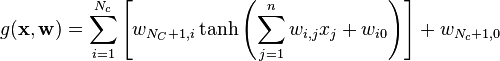# Difference between revisions of "Artificial neural network potentials"

Artificial neural network potentials (ANNP). Neural networks (NN) are used more and more for a wide array of applications. Here we are concerned with a more narrow application; their use in fitting   to an atomic or molecular potential energy surface. In particular the output layer, or node, provides an energy as a function of the coordinates, which form the input layer.

## Example

The output of a feedforward NN, having a single layer of hidden neurons, each having a sigmoid activation function and a linear output neuron, is given by:$g(\mathbf{x},\mathbf{w}) = \sum_{i=1}^{N_c} \left[ w_{N_C+1,i} \tanh \left( \sum_{j=1}^n w_{i,j} x_j + w_{i0} \right) \right] + w_{N_c+1,0}$

## Applications

Since the early work of Blank et al.  ANNS have been sucessfully developed for water , Al3+ ions dissolved in water , aqueous NaOH solutions , gold nanoparticles  as well as many other systems .